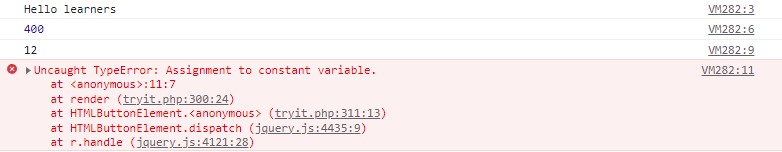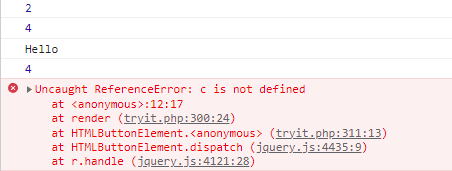GFG App
Open AppBrowser
Continue

Variables are the building blocks of any programming language. In JavaScript, a variable can be used to store reusable values. These values can be anything from Number, Boolean, String, and even a value returned from a function. The values of the variables are allocated using the assignment operator(“=”).

There are some basic rules to declare a variable in JavaScript:

• These are case-sensitive
• Can only begin with a letter, underscore(“_”) or “\$” symbol
• It can contain letters, numbers, underscore, or “\$” symbol
• A variable name cannot be a reserved keyword.

JavaScript is a dynamically typed language so the type of variables is decided at runtime. Therefore there is no need to explicitly define the type of a variable. We can declare variables in JavaScript in three ways:

Syntax:

```var geek = "Hello Geek"        // declaration using var
let \$ = "Welcome"                 // declaration using let
const _example = "Gfg"        // declaration using const   ```

All three keywords do the basic task of declaring a variable but with some differences Initially, all the variables in JavaScript were written using the var keyword but in ES6 the keywords let and const were introduced.

Example 1: In this example, we will declare variables using var

## Javascript

 `var` `a = ``"Hello Geeks"` `var` `b = 10;` `var` `c = 12;` `var` `d = b + c;` `console.log(a);` `console.log(b);` `console.log(c);` `console.log(d);`

Output:

```Hello Geeks
10
12
22```

Example 2: In this example, we will declare variables using let

## Javascript

 `let a = ``"Hello learners"` `let b = ``"joining"``;` `let c = ``" 12"``;` `let d = b + c;`   `console.log(a);` `console.log(b);` `console.log(c);` `console.log(d);`

Output

```Hello learners
joining
12
joining 12```

Example 3: In this example, we will declare the variable using the const keyword

## Javascript

 `const a = ``"Hello learners"` `console.log(a);`   `const b = 400;` `console.log(b);`   `const c = ``"12"``;` `console.log(c);`   `c = ``"new"` `console.log(c)`

Output:Explanation: const keyword is used when we assign a value permanently to a variable. So when we try to change the value of a variable declared with the const keyword it will throw an error. The variables declared with var and let are mutable that is their value can be changed but variables declared using const are immutable.

Note: The newly introduced keywords let and const are block scoped whereas var is function scoped.

Let us see an example to understand the difference:

Example:

## Javascript

 `{` `    ``let a = 2;` `    ``var` `b = 4` `    ``const c = ``"Hello"` `    ``console.log(a)` `    ``console.log(b)` `    ``console.log(c)` `}`   `console.log(b)` `console.log(c)` `console.log(a`

Output:Explanation: Since variables “a” and “c” are block scoped so we were not able to access them outside their block.